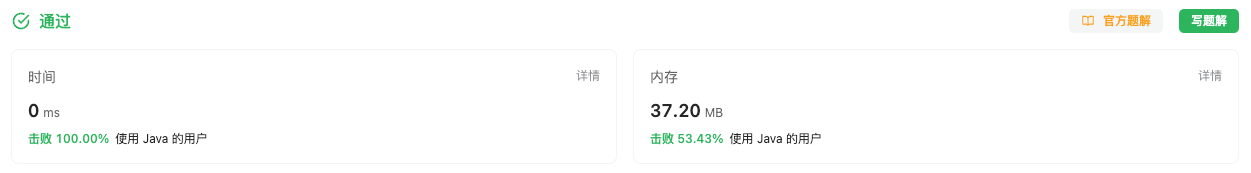#### 2582. 递枕头

###### 来源: 每日一题 2023.09.26

`n` 个人站成一排，按从 `1``n` 编号。

• 例如，当枕头到达第 `n` 个人时，TA 会将枕头传递给第 `n - 1` 个人，然后传递给第 `n - 2` 个人，依此类推。

``````输入：n = 4, time = 5

5 秒后，枕头传递到第 2 个人手中。
``````

``````输入：n = 3, time = 2

2 秒后，枕头传递到第 3 个人手中。
``````

• `2 <= n <= 1000`
• `1 <= time <= 1000`
``````class Solution {
public int passThePillow(int n, int time) {

}
}
``````

#### 分析与题解

• 模拟法

这个题目其实非常简单, 我们直接使用模拟法即可.

不管你走了多少秒, 枕头一定会在 `n` 个人中传递, 所以, 我们先把所有 `1 → n → 1` 这样的大循环去除, 剩余秒数我们一定是从 `1` 号开始,并且一定不能够再回到 `1` 了.

所以我们需要首先计算出去除 所有的 `1 → n → 1` 的循环, 还剩余的秒数. 其中 `1 → n` 需要 `n-1` 秒, 同样的 `n → 1` 也需要 `n-1` 秒.

``````int sub = time % ((n-1) * 2);
``````

这时候, 需要分为两种情况.

• 一种是从 `1` 出发, 没有从 `n` 返回.
``````return sub + 1;
``````
• 另外一种则是从 `n` 返回 `1` 的情况.
``````return n - (sub - (n-1));
``````

最后, 我们一起看一下这道简单题目的解题过程.

``````class Solution {
public int passThePillow(int n, int time) {
int sub = time%((n-1) * 2);
if (sub > n - 1) {
return n - (sub - (n-1));
} else {
return sub + 1;
}
}
}
``````

复杂度分析:

• 时间复杂度: O(1), 常量级别的时间复杂度.
• 空间复杂度: O(1), 常量级别的空间复杂度.

结果如下所示.IT界无底坑洞栋主 欢迎加Q骚扰:676758285